Foxtable(狐表)用户栏目专家坐堂 → [求助] 增加多行并赋值（部分列值不一样）

共有60人关注过本帖树形打印复制链接

主题：[求助] 增加多行并赋值（部分列值不一样）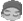by0521
1楼 | 信息 | 搜索 | 邮箱 | 主页 | UC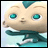加好友发短信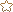[求助] 增加多行并赋值（部分列值不一样）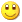Post By：2019/10/10 11:47:00 [只看该作者]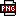此主题相关图片如下：qq图片20191010114300.png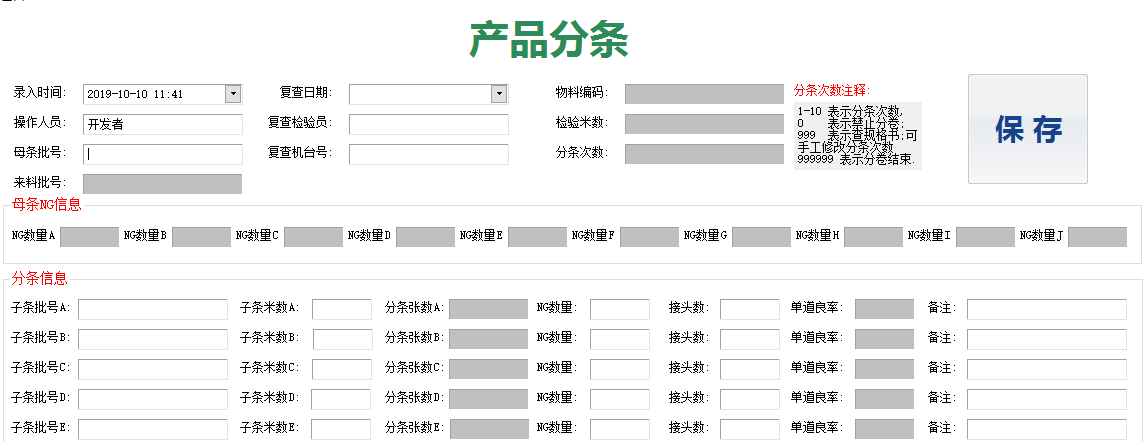根据分条次数来确定增加多少行，新增行的数据 上半部分的数据是一样的，代码可以这么写：For i As Integer = 1 To ftcs    Dim nr As Row = Tables("分条").AddNew    nr("录入时间") = e.Form.Controls("DateTimePicker3").Text    nr("操作人员") = e.Form.Controls("TextBox36").Text    nr("母条批号") = e.Form.Controls("TextBox1").Text    nr("来料批号") = e.Form.Controls("TextBox2").Text    nr("复查日期") = e.Form.Controls("DateTimePicker2").Text    nr("复查检验员") = e.Form.Controls("TextBox6").Text    nr("复查机台号") = e.Form.Controls("TextBox7").TextNext主要是下半部分的分条信息。每个都不一样。代码如何赋值正确？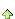2楼 | 信息 | 搜索 | 邮箱 | 主页 | UC加好友发短信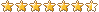Post By：2019/10/10 11:54:00 [只看该作者]

 控件名称定义的有规律一点，比如批号A控件名称为：TextBox_批号_1，米数A控件名称为：TextBox_米数_1，批号B控件名称为：TextBox_批号_2，米数B控件名称为：TextBox_米数_2，然后For i As Integer = 1 To ftcs    Dim nr As Row = Tables("分条").AddNew    nr("录入时间") = e.Form.Controls("DateTimePicker3").Text    nr("操作人员") = e.Form.Controls("TextBox36").Text    nr("母条批号") = e.Form.Controls("TextBox1").Text    nr("来料批号") = e.Form.Controls("TextBox2").Text    nr("复查日期") = e.Form.Controls("DateTimePicker2").Text    nr("复查检验员") = e.Form.Controls("TextBox6").Text    nr("复查机台号") = e.Form.Controls("TextBox7").Text    nr("子条批号") = e.Form.Controls("TextBox_批号_" & i).Text    nr("子条米数") = e.Form.Controls("TextBox_米数_" & i).TextNext